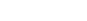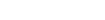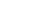AP Calculus: The Difference between a Graph and a Function?

Did you know that a graph of a function f is not the same as the function itself?

It might seem like there is clearly a difference, but sometimes it’s hard to articulate into words.

We have spoken about the definition of a function. Simply put, it’s a rule that transforms one real number into another real number. A graph is a geometric representation of that rule.

A Graph is a set.

If this is true, then according to the definition of a set, a graph is an unordered collection of objects. For this lesson, you need to know a little more about sets: the Cartesian product of two sets A and B is again a set, denoted  A x B and read “A cross B.” It is the set of all elements of the form (a, b) withThe Cartesian product is named after the famous French philosopher René Descartes:The points in a graph

The objects in the graph of a function are pointsordered pairs of real numbers in the Cartesian product of the set of real numbers with itself.  We call these points Cartesian coordinates.

We represent these points geometrically in what is known as the Cartesian plane, or simply the plane:The center of the plane, the point (0, 0) is called the origin, the horizontal axis is called the x-axis while the vertical axis is known as the y-axis. The two axes naturally divide the plane into quadrants. If a point (x, y) has both x, y positive, we say that (x, y) lies in the first quadrant; if (x, y) has x negative and y positive, then (x, y) lies in the second quadrant, etc.

The graph of a function

Given a function f whose domain is the set of real numbers and whose codomain is the set of real numbers, we say that the graph is the set of all points in the setof the form (x, f(x)) where x is a point in the domain of f.

Therefore the graph is a set that is unique for a given function, which geometrically represents the function. We can often use the graph of a function in order to deduce properties of said function.

Conclusion

The graph of a function and a function are closely related but NOT the same. Therefore when you are explaining a solution to a problem, make sure that you use “the function” and “the graph of the function” in the right places, depending on which you really mean.

Improve your SAT or ACT score, guaranteed. Start your 1 Week Free Trial of Magoosh SAT Prep or your 1 Week Free Trial of Magoosh ACT Prep today!Author

•In addition to blogging about AP Calculus, Oakland resident Chris Wirick has played the oboe in Beijing and Berlin, studied math and cognitive science (the other CS) at Cal Berkeley, and can’t stop gardening, cooking and eating new foods.